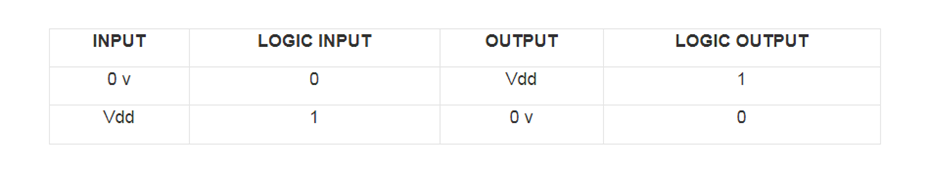top of page

# Introduction to CMOS

Updated: Sep 9Introduction to CMOS

Stands for "Complementary Metal Oxide Semiconductor." It is a technology used to produce integrated circuits. CMOS circuits are found in several types of electronic components, including microprocessors, batteries, and digital camera image sensors.

The "MOS" in CMOS refers to the transistors in a CMOS component, called MOSFETs (metal-oxide semiconductor field-effect transistors). The "metal" part of the name is a bit misleading, as modern MOSFETs often use poly-silicon instead of aluminum as the conductive material. Each MOSFET includes two terminals ("source" and "drain") and a gate, which is insulated from the body of the transistor. When enough voltage is applied between the gate and body, electrons can flow between the source and drain terminals.

The "complementary" part of CMOS refers to the two different types of semiconductors each transistor contains — N-type and P-type. N-type semiconductors have a greater concentration of electrons than holes or places where an electron could exist. P-type semiconductors have a greater concentration of holes than electrons. These two semiconductors work together and may form logic gates based on how the circuit is designed.

### CMOS Working Principle

• In CMOS technology, both N-type and P-type transistors are used to design logic functions. The same signal which turns ON a transistor of one type is used to turn OFF a transistor of the other type. This characteristic allows the design of logic devices using only simple switches, without the need for a pull-up resistor.

• In CMOS, logic gates a collection of n-type MOSFETs is arranged in a pull-down network between the output and the low voltage power supply rail (Vss or quite often ground). Instead of the load resistor of NMOS logic gates, CMOS logic gates have a collection of p-type MOSFETs in a pull-up network between the output and the higher-voltage rail (often named Vdd).

• Thus, if both a p-type and n-type transistor have their gates connected to the same input, the p-type MOSFET will be ON when the n-type MOSFET is OFF, and vice-versa. The networks are arranged such that one is ON and the other OFF for any input pattern as shown in the figure below.CMOS Working Principle

### CMOS Inverter

CMOS offers relatively high speed, low power dissipation, high noise margins in both states, and will operate over a wide range of source and input voltages (provided the source voltage is fixed). Furthermore, for a better understanding of the Complementary Metal Oxide Semiconductor working principle, we need to discuss in brief about CMOS logic gates as explained below. The inverter circuit as shown in the figure below. It consists of PMOS and NMOS FET. The input A serves as the gate voltage for both transistors.

CMOS InverterThe NMOS transistor has input from Vss (ground) and the PMOS transistor has input from Vdd. The terminal Y is output. When a high voltage (~ Vdd) is given at input terminal (A) of the inverter, the PMOS becomes open circuit and NMOS switched OFF so the output will be pulled down to Vss. When a low-level voltage (<Vdd, ~0v) applied to the inverter, the NMOS switched OFF and PMOS switched ON. So the output becomes Vdd or the circuit is pulled up to Vdd.CMOS Inverter

### The truth table of INVERTER is given in below tabletruth table of INVERTER

### CMOS NAND Gate

The below figure shows a 2-input Complementary MOS NAND gate. It consists of two series NMOS transistors between Y and Ground and two parallel PMOS transistors between Y and VDD.CMOS NAND GateIf either input A or B is logic 0, at least one of the NMOS transistors will be OFF, breaking the path from Y to Ground. But at least one of the pMOS transistors will be ON, creating a path from Y to VDD. Hence, the output Y will be high. If both inputs are high, both of the nMOS transistors will be ON and both of the pMOS transistors will be OFF. Hence, the output will be logic low.CMOS NAND Gate

### The truth table of the NAND logic gate given in below tabletruth table of the NAND logic gate

### CMOS NOR Gate

A 2-input NOR gate is shown in the figure below. The NMOS transistors are in parallel to pull the output low when either input is high. The PMOS transistors are in series to pull the output high when both inputs are low, as given in the below table. The output is never left floating.CMOS NOR Gate

### The truth table of NOR logic gate is given in the below table.Truth table NOR logic gate

• Very low static power consumption

• Reduce the complexity of the circuit

• The high density of logic functions on a chip

• Low static power consumption

• High noise immunity